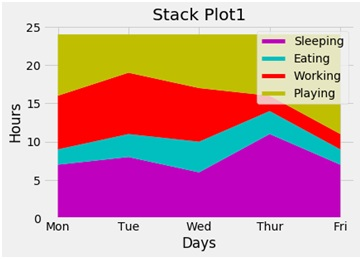# Python | Create stack plot using matplotlib.pyplot

Here, we are implementing a python program to create a stack plot using matplotlib.pyplot.
Submitted by Ayush Sharma, on November 25, 2018

Problem statement: Create stack plot in python (using matplotlib.pyplot).

Program:

```import matplotlib.pyplot as plt

days=[1,2,3,4,5]

sleeping = [7,8,6,11,7]
eating = [2,3,4,3,2]
working = [7,8,7,2,2]
playing = [8,5,7,8,13]

plt.plot([],[],color ='m', label = 'Sleeping', linewidth = 5)
plt.plot([],[],color ='c', label = 'Eating', linewidth = 5)
plt.plot([],[],color ='r', label = 'Working', linewidth = 5)
plt.plot([],[],color ='y', label = 'Playing', linewidth = 5)

plt.stackplot(days, sleeping, eating, working, playing, colors = ['m','c','r','y'])

plt.xlabel('Days')
plt.ylabel('Hours')
plt.title('Stack Plot1')
plt.xticks(days, ('Mon', 'Tue', 'Wed', 'Thur', 'Fri'))

plt.legend()
plt.show()
```

OutputExplanation:

Stackplot function is used to draw stackplot in python. The xlable, ylabel title gives the labels and title to the plot. Different colors can be given to the different levels of the stack plot.

Preparation